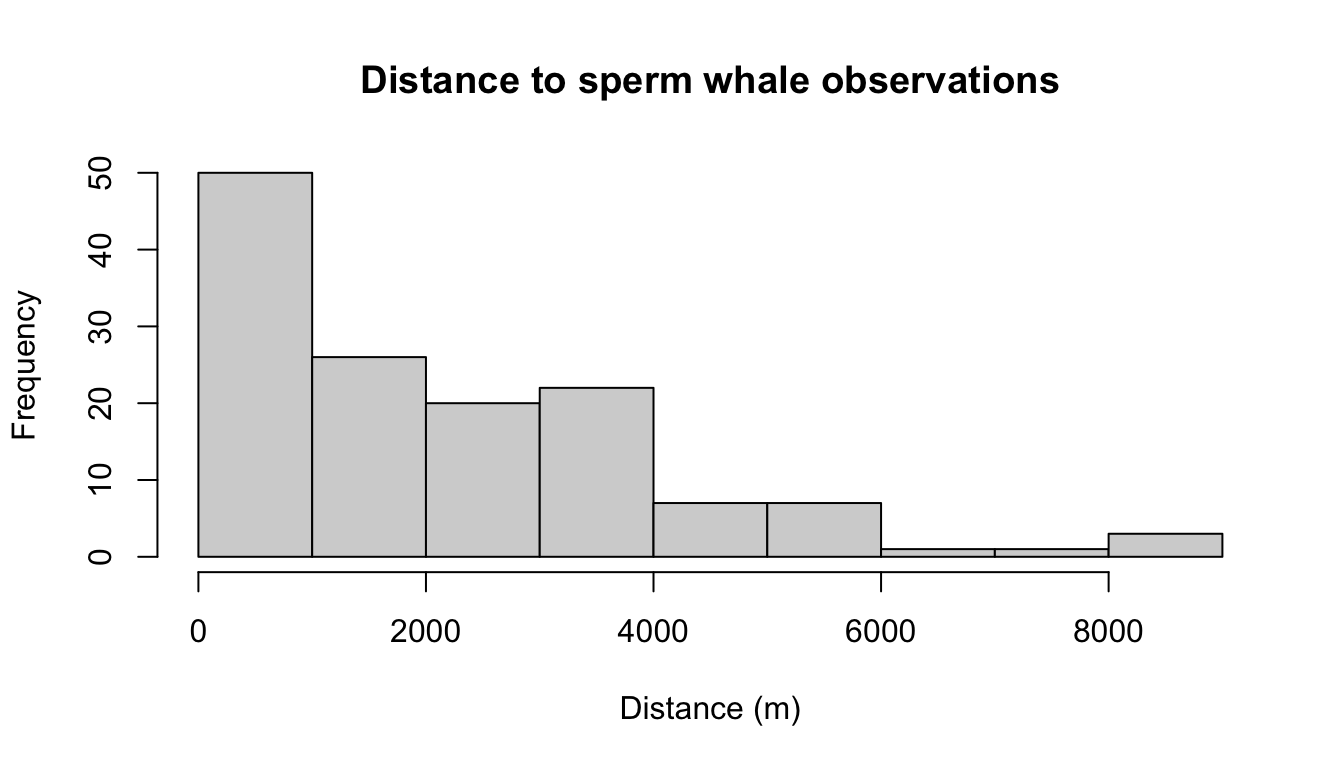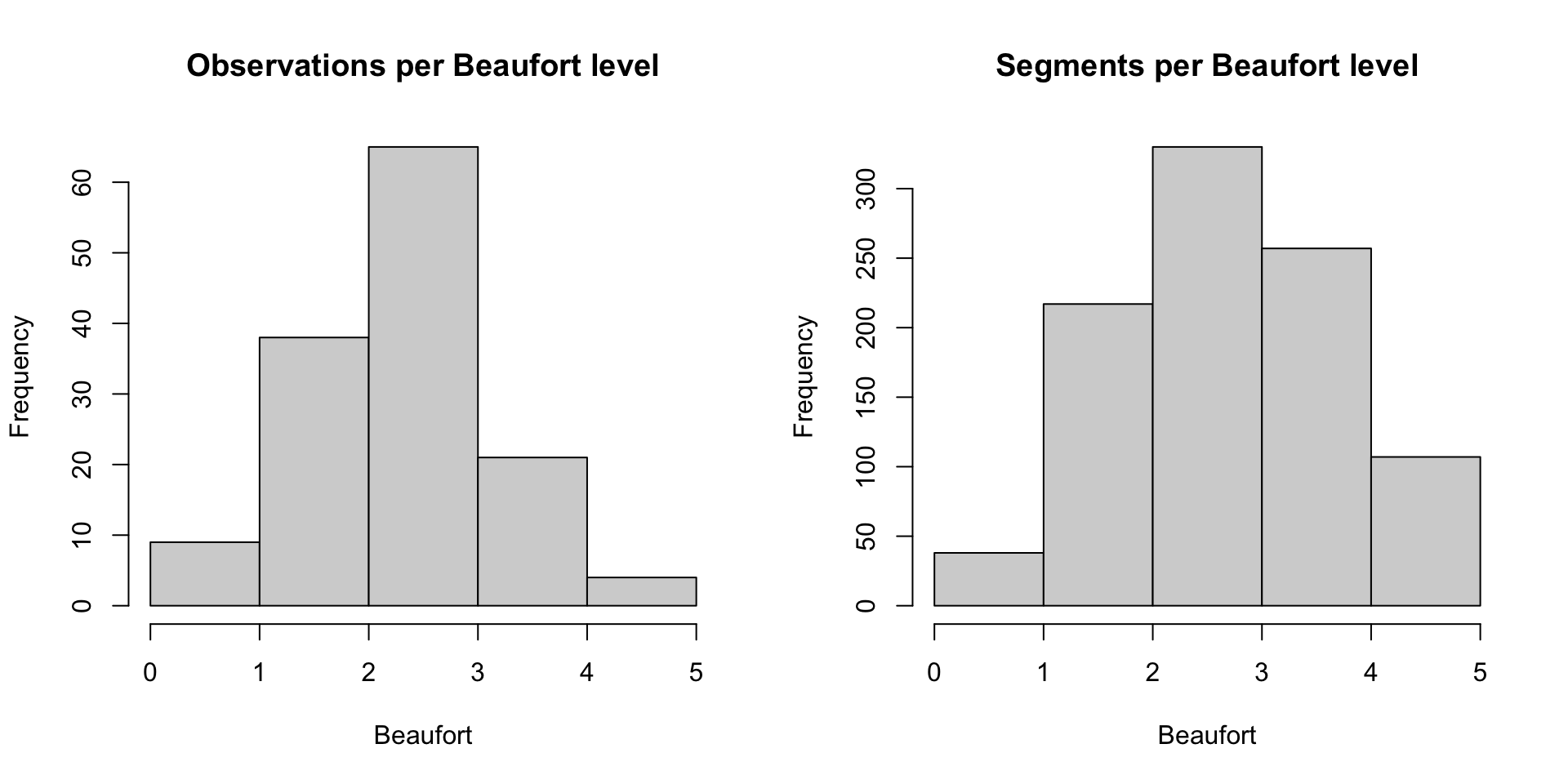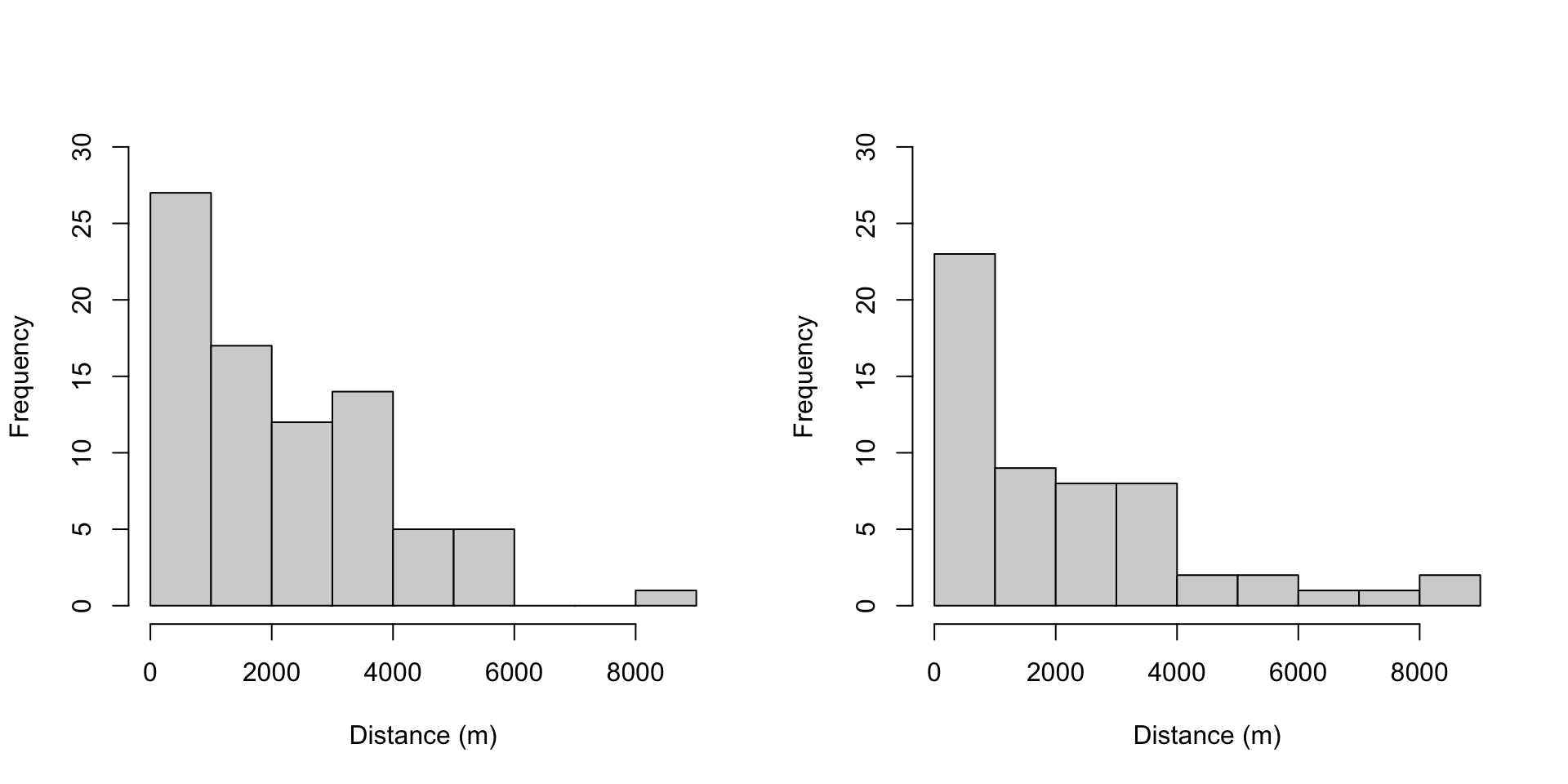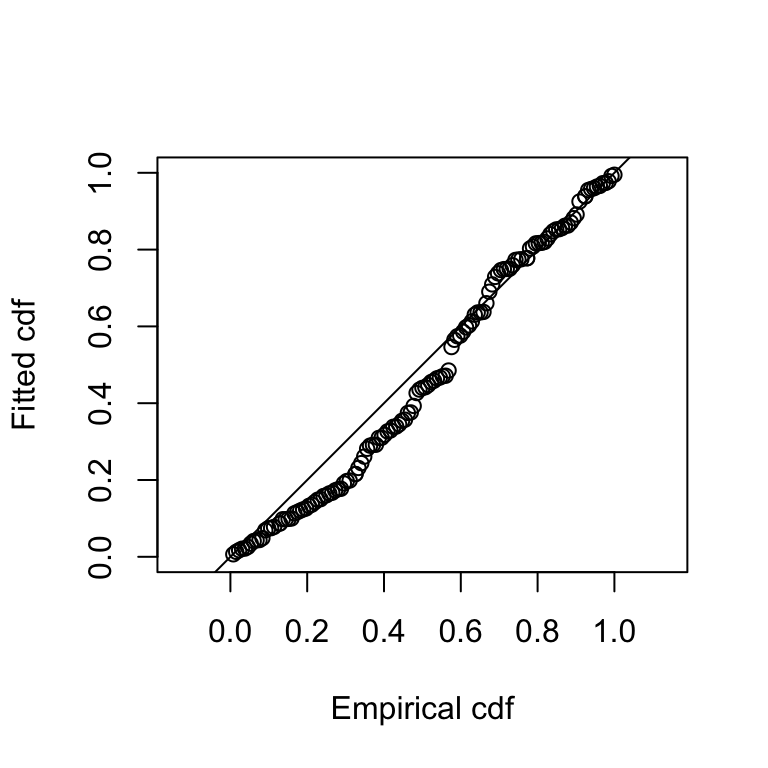## Aims

By the end of this practical you should feel confident doing the following:

• Fitting a detection function using ds()
• Checking detection functions using functions in package Distance
• Selecting models using AIC
• Estimating abundance (using R and maths!)

Solutions are given between these horizontal lines with bold headers

The “solutions” presented here are not definitive, many modelling options are possible. Our aim in this practical is to have some detection functions fitted to use later on and have you get familiar with the Distance package.

## Preamble

First need to load the requisite R libraries

library(Distance)
## Loading required package: mrds
## This is mrds 2.2.3
## Built: R 4.0.2; ; 2020-08-01 10:33:56 UTC; unix
##
## Attaching package: 'Distance'
## The following object is masked from 'package:mrds':
##
##     create.bins
library(ggplot2)
library(knitr)

We can then load the data from the RData file (this needs to be in your R working directory):

load("spermwhale.RData")

## Exploring the data

Before setting off fitting detection functions, let’s look at the relationship of various variables in the data.

The most important covariate in a distance sampling analysis is distance itself. We can plot a histogram of the distances to check that (1) we imported the data correctly and (2) it conforms to the usual shape for line transect data.

hist(distdata$distance, xlab="Distance (m)", main="Distance to sperm whale observations")Distribution of observed perpendicular detection distances. We can also see how many observations were recorded per Beaufort level (some background on Beaufort with photos). We can first use table() to see what values occur and how many there are: table(distdata$Beaufort)
##
##   0   1 1.8   2 2.4 2.5 2.7 2.8 2.9   3 3.1 3.5 3.6 3.7 3.9   4 4.5   5
##   5   4   7  31   8  10   1   1   4  41   2   6   1   2   2   8   1   3

The survey protocol on these surveys took an average of several observers’ assessments of the weather, hence we get these decimal values. We can use the breaks= argument to hist() to bin these values to integer values. We’ll plot the values from the segments at the same time, so we can get an idea of what the conditions were like over the whole survey, not just when we saw sperm whales:

par(mfrow=c(1,2))

hist(distdata$Beaufort, breaks=seq(0, 5, by=1), main="Observations per Beaufort level", xlab="Beaufort") hist(segs$Beaufort, breaks=seq(0, 5, by=1),
main="Segments per Beaufort level",
xlab="Beaufort")We can see here that most of the segments had a Beaufort >= 2 and very few had Beaufort of 0-1 (right plot). We can see then that there are few observations at the lowest Beaufort (left plot) because there just wasn’t much time to observe things in those conditions. We see that with increasing Beaufort observations fall off (left plot), even as there were plenty of segments with those higher Beaufort levels (right plot).

We can create a rounded version of the variable using the cut function in R for later:

distdata$BeaufortCut <- cut(distdata$Beaufort, seq(0, 5, by=1),
include.lowest=TRUE,
ordered.result=TRUE)

Given we are including data from two different surveys we can also check that the surveys have similar histograms of distance (we’ll check with the detection function below whether we need to include a covariate to control for this).

par(mfrow=c(1,2))

hist(subset(distdata, Survey=="en04395")$distance, main="", ylim=c(0, 30), xlab="Distance (m)") hist(subset(distdata, Survey=="GU0403")$distance,
main="", ylim=c(0, 30),
xlab="Distance (m)")## Fitting detection functions

It’s now time to fit some detection function models. We’ll use the ds() function from the Distance package to fit the detection function. You can access the help file for the ds() function by typing ?ds – this will give you information about what the different arguments to the function are and what they do.

We can fit a very simple model with the following code:

df_hn <- ds(data=distdata, truncation=6000, key="hn", adjustment=NULL)
## Fitting half-normal key function
## Key only model: not constraining for monotonicity.
## AIC= 2252.06
## No survey area information supplied, only estimating detection function.

Let’s dissect the call and see what each argument means:

• data=distdata: the data to use to fit the model, as we prepared above.
• truncation=6000: set the truncation distance. Here, observations at distances greater than 6000m will be discarded before fitting the detection function.
• key="hn": the key function to use for the detection function, in this case half-normal (?ds lists the other options).
• adjustment=NULL: adjustment term series to fit. NULL here means that no adjustments should be fitted (again ?ds lists all options).

Other useful arguments for this practical are:

• formula=: gives the formula to use for the scale parameter. By default it takes the value ~1, meaning the scale parameter is constant and not a function of covariates.
• order=: specifies the “order” of the adjustments to be used. This is a number (or vector of numbers) specifying the order of the terms. For example order=2 fits order 2 adjustments, order=c(2,3) will fit a model with order 2 and 3 adjustments (mathematically, it only makes sense to include order 3 with order 2). By default the value is NULL which has ds() select the number of adjustments by AIC.

We set truncation=6000 here, why? The truncation removes observations in the tail of the distribution. We do this because we only care about the detection function near 0, that has the most effect on $$\hat{p}$$ (since it’s where the detection function is biggest). We need to trade-off between getting the shape right and not using lots of parameters modelling what’s going on far from the observer and has little effect on the result. Here we use ~96% of the data. Len Thomas suggests $$g(w)\approx 0.15$$ (i.e., at the truncation distance the detection function should be about 0.15). You can play around with this if you’re interested here by changing truncation= and seeing what the differences in $$\hat{p}$$ are (but push on to the next section if you’re happy!).

Trying to fit a few detection functions

First we can try using a hazard-rate detection function:

df_hr <- ds(data=distdata, truncation=6000, key="hr", adjustment=NULL)
## Fitting hazard-rate key function
## Key only model: not constraining for monotonicity.
## AIC= 2247.594
## No survey area information supplied, only estimating detection function.

We can also try out using covariates in the detection function. Sea state (as we discretized above), using a hazard-rate and half-normal key function:

df_hn_beau <- ds(data=distdata, truncation=6000, key="hn",
adjustment=NULL, formula=~BeaufortCut)
## Fitting half-normal key function
## AIC= 2236.726
## No survey area information supplied, only estimating detection function.
df_hr_beau <- ds(data=distdata, truncation=6000, key="hr",
adjustment=NULL, formula=~BeaufortCut)
## Fitting hazard-rate key function
## AIC= 2240.354
## No survey area information supplied, only estimating detection function.

We can also include the observed group size:

df_hn_beau_size <- ds(data=distdata, truncation=6000, key="hn",
adjustment=NULL, formula=~BeaufortCut+size)
## Fitting half-normal key function
## AIC= 2238.411
## No survey area information supplied, only estimating detection function.
df_hr_beau_size <- ds(data=distdata, truncation=6000, key="hr",
adjustment=NULL, formula=~BeaufortCut+size)
## Fitting hazard-rate key function
## AIC= 2242.175
## No survey area information supplied, only estimating detection function.

Again this is just a sampling of the possible models we could fit to the detectability data. Next we’ll inspect these models…

### Summaries

We can look at the summary of the fitted detection function using the summary() function:

summary(df_hn)
##
## Summary for distance analysis
## Number of observations :  132
## Distance range         :  0  -  6000
##
## Model : Half-normal key function
## AIC   : 2252.06
##
## Detection function parameters
## Scale coefficient(s):
##             estimate         se
## (Intercept) 7.900732 0.07884776
##
##                        Estimate          SE         CV
## Average p             0.5490484  0.03662569 0.06670757
## N in covered region 240.4159539 21.32287580 0.08869160

Looking at summaries

I won’t go through all of the above here, but just compare with and without covariates, let’s compare summary(df_hn) output above to that for

summary(df_hr_beau_size)
##
## Summary for distance analysis
## Number of observations :  132
## Distance range         :  0  -  6000
##
## Model : Hazard-rate key function
## AIC   : 2242.175
##
## Detection function parameters
## Scale coefficient(s):
##                     estimate        se
## (Intercept)       8.59365686 1.0118407
## BeaufortCut(1,2] -1.24495135 1.0240637
## BeaufortCut(2,3] -0.34995758 0.9789634
## BeaufortCut(3,4] -1.57939745 1.0583225
## BeaufortCut(4,5] -3.64460160 1.3577675
## size             -0.07723057 0.1353780
##
## Shape coefficient(s):
##              estimate        se
## (Intercept) 0.5318624 0.2700877
##
##                      Estimate          SE        CV
## Average p             0.36359  0.09128592 0.2510683
## N in covered region 363.04629 95.41676384 0.2628226

Note the difference in the Average p between the two models. In the covariate case, this is averaged over the detection probabilities for the different covariate values. We can look at the distribution of the probabilities of detection for each observation using the p_dist_table function:

p_dist_table(df_hr_beau_size)
##          p count
##    0 - 0.1     4
##  0.1 - 0.2     0
##  0.2 - 0.3    21
##  0.3 - 0.4    38
##  0.4 - 0.5     0
##  0.5 - 0.6     1
##  0.6 - 0.7    26
##  0.7 - 0.8    38
##  0.8 - 0.9     4
##    0.9 - 1     0
## Range of probabilities:  0.045 - 0.84

We can see there’s a broad range of probabilities of detection estimated for this model that get averaged out in the above summary output. Always worth checking!

### Goodness of fit

Goodness of fit quantile-quantile plot and test results can be accessed using the gof_ds() function:

gof_ds(df_hn)Goodness of fit QQ plot of half-normal detection function.

##
## Goodness of fit results for ddf object
##
## Distance sampling Cramer-von Mises test (unweighted)
## Test statistic = 0.396179 p-value = 0.0739475

Comparing Q-Q plots

Let’s run gof_ds() on each of our models and look at the corresponding plots arranged in a grid

# make a grid, 3 rows by 2 columns
par(mfrow=c(3,2))

gof_ds(df_hn, main="df_hn")
##
## Goodness of fit results for ddf object
##
## Distance sampling Cramer-von Mises test (unweighted)
## Test statistic = 0.396179 p-value = 0.0739475
gof_ds(df_hr, main="df_hr")
##
## Goodness of fit results for ddf object
##
## Distance sampling Cramer-von Mises test (unweighted)
## Test statistic = 0.0453519 p-value = 0.903832
gof_ds(df_hn_beau, main="df_hn_beau")
##
## Goodness of fit results for ddf object
##
## Distance sampling Cramer-von Mises test (unweighted)
## Test statistic = 0.170184 p-value = 0.333529
gof_ds(df_hr_beau, main="df_hr_beau")
##
## Goodness of fit results for ddf object
##
## Distance sampling Cramer-von Mises test (unweighted)
## Test statistic = 0.0451092 p-value = 0.905226
gof_ds(df_hn_beau_size, main="df_hn_beau_size")
##
## Goodness of fit results for ddf object
##
## Distance sampling Cramer-von Mises test (unweighted)
## Test statistic = 0.166067 p-value = 0.344127
gof_ds(df_hr_beau_size, main="df_hr_beau_size")